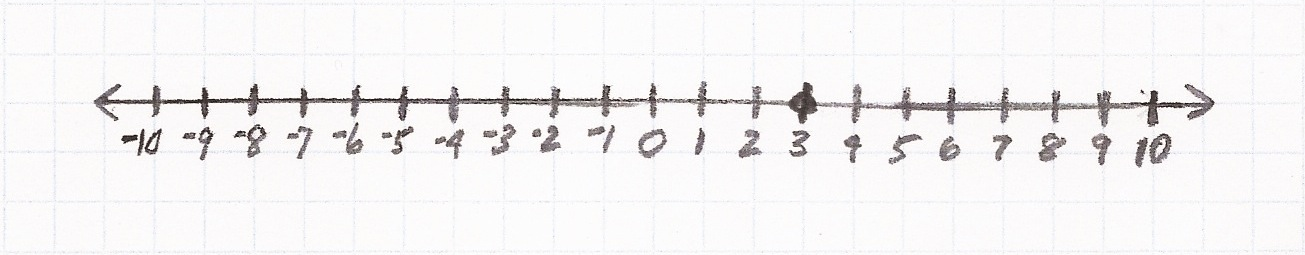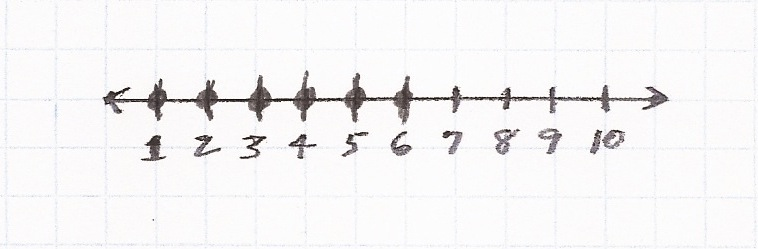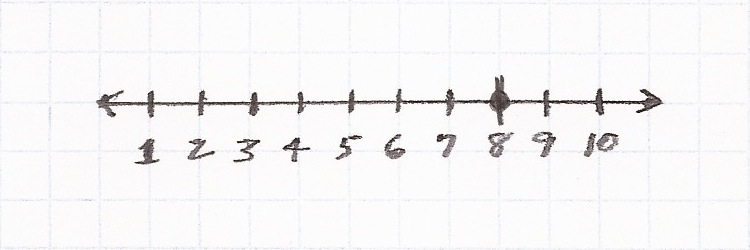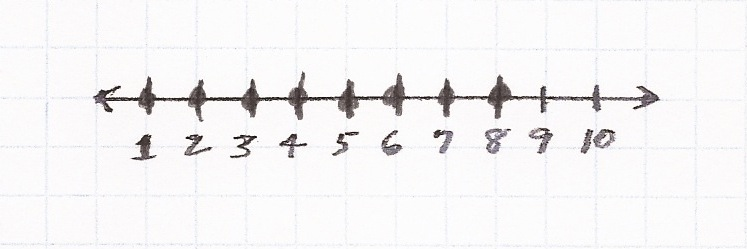# Number LineBasics
Number Line refers to a series of points, usually representing whole numbers, extending infinitely on a line.

Number Line showing both positive and negative numbers.Number Line showing positive numbers.Question

Plot point 3 on the number line.Question
Plot point -3 on the number line.Question
Plot all whole numbers from -3 through 3 on the number line.Question
Plot all whole numbers from 1 through 6 on the number line.Practice – Questions
1.  Plot 4 on the number line.3.  Plot all whole numbers -7 through 4 on the number line.5.  Plot all whole numbers from 1 through 8 on the number line.1.2.3.4.5.